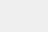# 为了这个羞羞的机器学习项目，我差点成为“鉴黄师”https://www.autoblow.com/bjpaper/

## 数据集## 动作分析## K均值聚类算法## 程序生成## 马尔可夫链模型### Dense Neural Network (DNN) ModelDNN Sequence

## 模型比较[ 2 * frac{sum_{s=1}^{16} sum_{i=1}^{16} n_{is} * (1 – p_{is})}{sum_{s=1}^{16} sum_{i=1}^{16} n_{is}} ]

## 未来的研究点击下方 |  | 了解更多